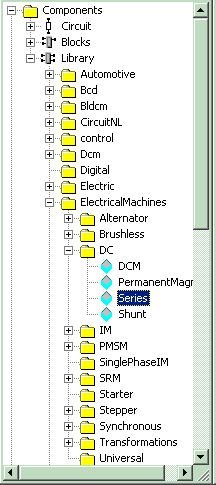• Introduction
• What is in this manual
• What is Caspoc
• User interface
• Introduction
• Starting
• Simulation
• Editing
• Viewing and printing
• Getting Started
• Basic editing
• Simulation in the time domain
• Basic User Interface Topics
• Editing
• Simulation
• Viewing
• Library
• Reports
• Project management
• Circuit and Block Diagram Components
• Introduction
• Cscript and user defined functions
• Component parameters
• Modeling Topics
• Introduction
• Power Electronics
• Semiconductors
• Electrical Machines
• Electrical drives
• Power Systems
• Mechanical Systems
• Thermal Systems
• Magnetic Circuits
• Green Energy
• Coupling to FEM
• Experimenter
• Analog hardware description language
• Embedded C code Export
• Coupling to Spice
• Small Signal Analysis
• Matlab coupling
• Tips and tricks
• Appendices

## Creating a DC drive.

The DC Drive is a simple system to model. In the library you will find various electrical machines. From the library we will select a Series DC machine and connect it to a voltage source and a mechanical load. The Series DC machine is selected from components/library/ElectricalMachines/DCConnect a voltage source, that i1s selected from components/circuit/sources, of 10 volts to the electrical terminals of the DC machine. The mechanical axis of the machine is connected to an inertia that is selected from components/circuit/rotational. A bearing that is selected from components/circuit/rotational is connected to he axis. You can draw the axis just like drawing electrical wires.

The parameters for the electrical machine are default and are

• Ra=0.07
• Rf=0.07
• La=5m
• Lf=5m
• Jm=2m
• K=0.256
The parameters for the mechanical load are
• J=1m
• f=10m
The total simulation time is set to 1 second to see the start up of the machine. The mechanical time constant of the machine is tau=(Jrotor+Jaxis)/f. The time step for the simulation is set to 1msSince the mechanical time constant of the DC machine is larger than the electrical time constant (La+LF)/(Ra+Rf), the start up of the electrical machine is dependent on the total inertia of the rotor and load and the friction in the bearing.
The results from the simulation are displayed in the scope. Notice that the current measurement in the voltage source is the current flowing into the voltage source and is therefore negative.The red trace is the angular speed of the axis and the red trace is the current flowing into the DC voltage source.